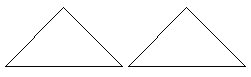Name: ___________________Date:___________________

 Email us to get an instant 20% discount on highly effective K-12 Math & English kwizNET Programs!

### Grade 3 - Mathematics8.9 Congruent and Symmetric Figures

 When two figures are exactly the same, they are said to be congruent. When a figure can be folded into equal pieces, then it is said to be symmetric. The line along which the figure is folded is called the line of symmetry. Example: How many lines of symmetry does this figure have?Answer: 4 Directions: Answer the following. Also draw at least five symmetric figures and show their line of symmetry.
 Q 1: How many lines of symmetry does a circle have?101590more than you can count Q 2: In a symmetrical figure the folded line is calledradiusdiameterthe line of symmetry Q 3: How many lines of symmetry does a square have?3241 Q 4: Are the two triangles in the figure congruent? (hint: sometimes you have to turn the figure around to see if it is congruent with another)yesno Q 5: These two figers are congruent.FalseTrue Q 6: Are the two triangles in the figure congruent?FalseTrue Question 7: This question is available to subscribers only! Question 8: This question is available to subscribers only!

#### Subscription to kwizNET Learning System costs less than \$1 per month & offers the following benefits:

• Unrestricted access to grade appropriate lessons, quizzes, & printable worksheets
• Instant scoring of online quizzes
• Progress tracking and award certificates to keep your student motivated
• Unlimited practice with auto-generated 'WIZ MATH' quizzes